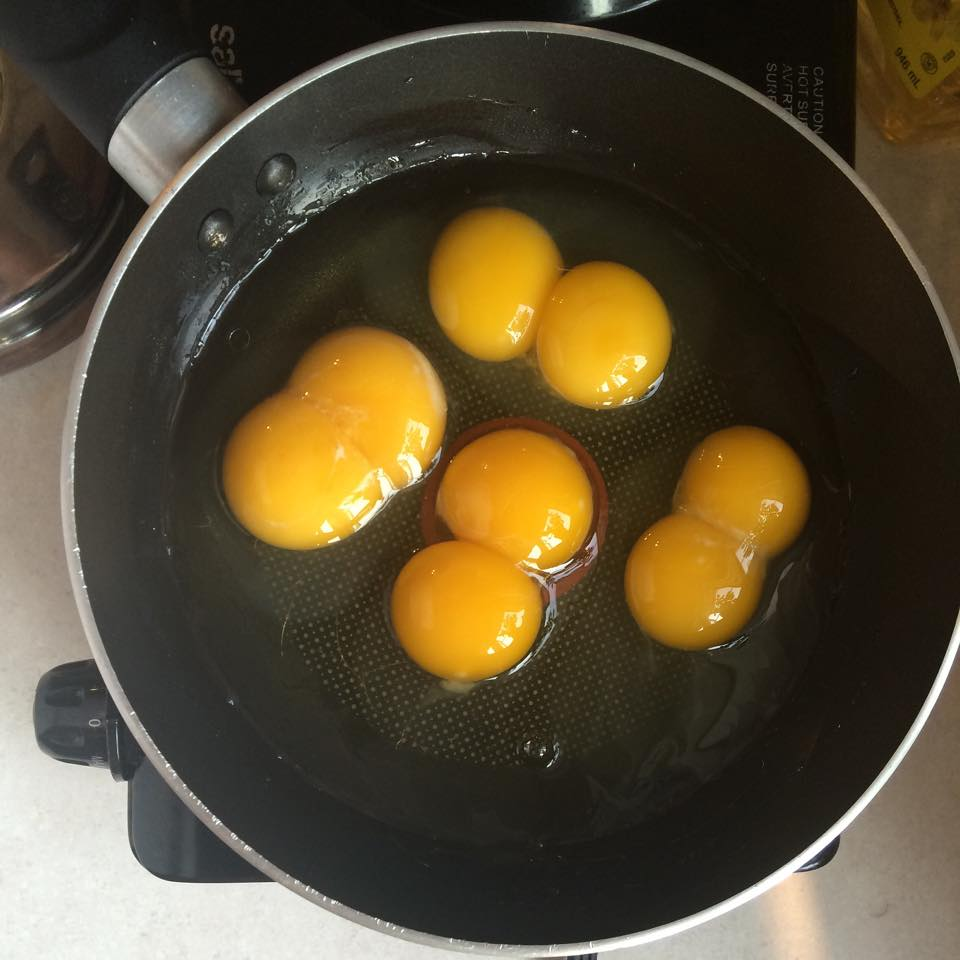On a sunny July 6th 2015, u/cheonfk cracked four eggs in a pan and got eight yolks. I then did a bunch of math to demonstrate why it’s always important to put unlikely odds into perspective.According to the British Egg Information Service about 0.1% of eggs have a double yolk. For the sake of the exercise we’ll assume this is uniform across all eggs1 and ignore yolkless and higher polyyolk eggs. That means there’ll be a 99.9% chance of getting a single yolk and we can then work out the probability that we have $$d$$ double yolks in a pan of four.

\begin{align} \mathbb{P}(d = 0) &= 1 \times {0.999}^4 \times {0.001}^0 = 99.6\% \\ \mathbb{P}(d = 1) &= 4 \times {0.999}^3 \times {0.001}^1 = 0.399\% \\ \mathbb{P}(d = 2) &= 6 \times {0.999}^2 \times {0.001}^2 = 0.000599\% \\ \mathbb{P}(d = 3) &= 4 \times {0.999}^1 \times {0.001}^3 = 0.000000400\% \\ \mathbb{P}(d = 4) &= 1 \times {0.999}^0 \times {0.001}^4 = 0.0000000001\% \\ \end{align}

With decimals like this it can be hard to comprehend how astronomically low the odds are so I’ll try to put them into perspective. Assuming you cracked 4 eggs in the pan every day you would expect to see

• 1 double yolk on average once every 251 days,
• 2 every 457 years (the time from now to Shakespeare),
• 3 every 685,000 years (now to Humans and Neanderthals diverging),2
• 4 doubles every 2.7 billion years.

That last one is from now all the way back to cyanobacteria being the only life on earth3, a pretty long time to wait. Let’s get some people along to speed the process up. You would need:

• 250 people doing it once each to get one double on average,
• every person in Guam (160k people) to do it once to get two doubles,
• every person in Indonesia (250m people) to do it once for three,
• and every person on Earth (7bn) to do it 143 times to get all four.

Seems like lot right? Let’s bring that back down and make it real. The UK egg consumption is estimated at 12 billion eggs per year or 0.5 eggs per person per day. Lets enforce an international law that requires people to cook their eggs only in batches of 4. Assuming every person on earth has this same consumption, that means that our 4 double yolk phenomena happens on average somewhere every

$\frac{4}{0.5} \times \frac{143}{365} \approx 3~\text{years.}$

As an extra tit-bit, since getting fewer than four polyyolks needs fewer than 7 billion people doing it once, on average they’d happen somewhere on Earth multiple times a day: 28 times per day for three doubles, 44000 per day for two doubles, and 28,000,000 for a single double yolk in four.

#### Conclusion

Multiple double yolks is a magic rare experience if you just look at it from the perspective of 1 person. But when you account for all 7,000,000,000 people on this brilliant egg filled planet (and institute international laws on egg cooking) the odds are not improbable.

1. As explained by this BBC article, egg yolkness is not independent. The probability of the first being double yolked is 0.1%, but if it is the probability that subsequent eggs are double yolked is about 1%.

2. Research that happened since I wrote this suggests that it happened at least 800,000 years ago.

3. The latest research suggests other simple life forms such as methanogens existed earlier than this.